# 力扣OJ（1401-1500）

1414. 和为 K 的最少斐波那契数字数目

1419. 数青蛙

1420. 生成数组

1424. 对角线遍历 II

1426. 数元素

1427. 字符串的左右移

1428. 至少有一个 1 的最左端列

1430. 判断给定的序列是否是二叉树从根到叶的路径

1441. 用栈操作构建数组

1442. 形成两个异或相等数组的三元组数目

1443. 收集树上所有苹果的最少时间

1450. 在既定时间做作业的学生人数

1451. 重新排列句子中的单词

1452. 收藏清单

1455. 检查单词是否为句中其他单词的前缀

1456. 定长子串中元音的最大数目

1457. 二叉树中的伪回文路径

1458. 两个子序列的最大点积

1461. 检查一个字符串是否包含所有长度为 K 的二进制子串

1464. 数组中两元素的最大乘积

1465. 切割后面积最大的蛋糕

1466. 重新规划路线

1467. 两个盒子中球的颜色数相同的概率

1470. 重新排列数组

1471. 数组中的 k 个最强值

1472. 设计浏览器历史记录

1473. 给房子涂色 III

1474. 删除链表 M 个节点之后的 N 个节点

1480. 一维数组的动态和

1481. 不同整数的最少数目

1482. 制作 m 束花所需的最少天数

1483. 树节点的第 K 个祖先

1486. 数组异或操作

1487. 保证文件名唯一

1488. 避免洪水泛滥

1489. 找到最小生成树里的关键边和伪关键边

1494. 并行课程 II

1496. 判断路径是否相交

# 1419. 数青蛙

1 <= croakOfFrogs.length <= 10^5

class Solution {
public:
int minNumberOfFrogs(string croakOfFrogs) {
map<char,int>m;
int ans=0;
for(int i=0;i<croakOfFrogs.length();i++)
{
m[croakOfFrogs[i]]++;
if(m['c']<m['r'] || m['r']<m['o'] || m['o']<m['a'] || m['a']<m['k'])return -1;
ans=max(ans,m['c']-m['k']);
}
if(m['c']>m['r'] || m['r']>m['o'] || m['o']>m['a'] || m['a']>m['k'])return -1;
return ans;
}
};

# 1424. 对角线遍历 II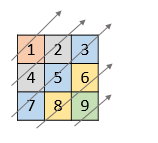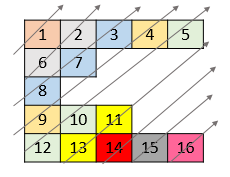1 <= nums.length <= 10^5
1 <= nums[i].length <= 10^5
1 <= nums[i][j] <= 10^9
nums 中最多有 10^5 个数字。

void f(vector<vector<int>>&ans, vector<int>&v)
{
vector<int>tmp;
ans.insert(ans.begin(), tmp);
for (int i = 0; i < v.size(); i++)
{
if (i >= ans.size())ans.push_back(tmp);
ans[i].push_back(v[i]);
}
}
class Solution {
public:
vector<int> findDiagonalOrder(vector<vector<int>>& nums) {
vector<vector<int>>ans;
for (int i = nums.size() - 1; i >= 0; i--)f(ans, nums[i]);
vector<int>res;
for (int i = 0; i < ans.size(); i++)
{
for (int j = 0; j < ans[i].size(); j++)res.push_back(ans[i][j]);
}
return res;
}
};

class Solution {
public:
vector<int> findDiagonalOrder(vector<vector<int>>& nums) {
map<int,vector<int>>ans;
vector<int>emp;
int k=-1;
for(int i=0;i<nums.size();i++)for(int j=0;j<nums[i].size();j++)
{
if(k<i+j)ans[++k]=emp;
ans[i+j].push_back(nums[i][j]);
}
vector<int>res;
for (int i = 0; i < ans.size(); i++)
{
for (int j = ans[i].size()-1;j>=0;j--)res.push_back(ans[i][j]);
}
return res;
}
};

# 1426. 数元素

1 <= arr.length <= 1000
0 <= arr[i] <= 1000

class Solution {
public:
int countElements(vector<int>& arr) {
map<int, int>m;
for (auto& ai : arr)m[ai]++;
int ans = 0;
for (auto& ai : arr)ans += m[ai + 1] ? 1 : 0;
return ans;
}
};

# 1427. 字符串的左右移

direction 可以为 0 （表示左移）或 1 （表示右移）。
amount 表示 s 左右移的位数。

[0,1] 表示左移 1 位。 "abc" -> "bca"
[1,2] 表示右移 2 位。 "bca" -> "cab"

[1,1] 表示右移 1 位。 "abcdefg" -> "gabcdef"
[1,1] 表示右移 1 位。 "gabcdef" -> "fgabcde"
[0,2] 表示左移 2 位。 "fgabcde" -> "abcdefg"
[1,3] 表示右移 3 位。 "abcdefg" -> "efgabcd"

1 <= s.length <= 100
s 只包含小写英文字母
1 <= shift.length <= 100
shift[i].length == 2
0 <= shift[i] <= 1
0 <= shift[i] <= 100

class Solution {
public:
string stringShift(string s, vector<vector<int>>& shift) {
int n = 0, len = s.length();
for (auto& si : shift)n += (si * 2 - 1) * si;
n = (n % len + len) % len;
return s.substr(len - n, n) + s.substr(0, len - n);
}
};


# 1428. 至少有一个 1 的最左端列

（这是一个交互题）

BinaryMatrix.get(row, col) 返回位于索引 (row, col) （从 0 开始）的元素。
BinaryMatrix.dimensions() 返回含有 2 个元素的列表 [rows, cols]，表示这是一个 rows * cols的矩阵。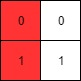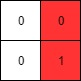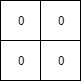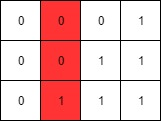rows == mat.length
cols == mat[i].length
1 <= rows, cols <= 100
mat[i][j] 只会是 0 或 1。
mat[i] 已按非递减顺序排序。

class Solution {
public:
int leftMostColumnWithOne(BinaryMatrix& bm) {
int a = 0, row = -1, col = bm.dimensions() - 1;
while (true) {
if (a == 0)row++;
else col--;
if (row >= bm.dimensions() || col < 0)break;
a = bm.get(row, col);
}
return col< bm.dimensions() - 1 ? col + 1 : -1;
}
};

# 1441. 用栈操作构建数组

Push：从 list 中读取一个新元素， 并将其推入数组中。
Pop：删除数组中的最后一个元素。

1 <= target.length <= 100
1 <= target[i] <= 100
1 <= n <= 100
target 是严格递增的

class Solution {
public:
vector<string> buildArray(vector<int>& target, int n) {
vector<string>ans;
int key=0;
for(auto it=target.begin();it!=target.end();it++)
{
int k=*it;
key++;
while(key<k)
{
key++;
ans.insert(ans.end(),"Push");
ans.insert(ans.end(),"Pop");
}
ans.insert(ans.end(),"Push");
}
return ans;
}
};

# 1442. 形成两个异或相等数组的三元组数目

a 和 b 定义如下：

a = arr[i] ^ arr[i + 1] ^ ... ^ arr[j - 1]
b = arr[j] ^ arr[j + 1] ^ ... ^ arr[k]

1 <= arr.length <= 300
1 <= arr[i] <= 10^8

class Solution {
public:
int countTriplets(vector<int>& arr) {
int len=arr.size();
int ans=0;
for(int i=1;i<len;i++)
{
arr[i]^=arr[i-1];
if(arr[i]==0)ans+=i;
}
for(int i=0;i<len;i++)for(int k=i+1;k<len;k++)
if(arr[i]==arr[k])ans+=k-i-1;
return ans;
}
};

# 1443. 收集树上所有苹果的最少时间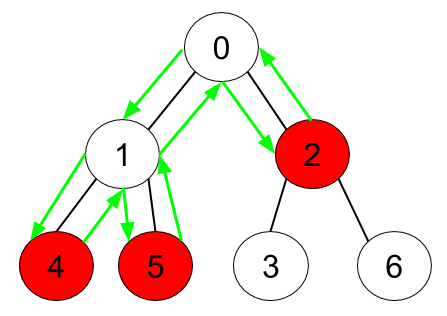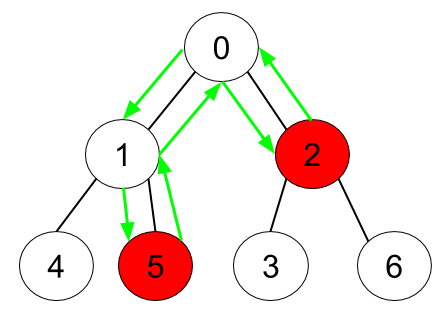1 <= n <= 10^5
edges.length == n-1
edges[i].length == 2
0 <= fromi, toi <= n-1
fromi < toi
hasApple.length == n

class Solution {
public:
int minTime(int n, vector<vector<int>>& edges, vector<bool>& hasApple) {
int *fa=new int[n];
int *ans=new int[n];
for(int i=0;i<n;i++)ans[i]=0;
ans=1;
for(auto it=edges.begin();it!=edges.end();it++)fa[(*it)]=(*it);
fa=0;
for(int i=0;i<n;i++)if(hasApple[i])
{
int j=i;
while(ans[j]==0)
{
ans[j]=1,j=fa[j];
}
}
int res=-1;
for(int i=0;i<n;i++)if(ans[i])res++;
return res*2;
}
};

# 1450. 在既定时间做作业的学生人数

startTime.length == endTime.length
1 <= startTime.length <= 100
1 <= startTime[i] <= endTime[i] <= 1000
1 <= queryTime <= 1000

class Solution {
public:
int busyStudent(vector<int>& startTime, vector<int>& endTime, int queryTime) {
int ans=0;
for(int i=0;i<startTime.size();i++)
if(startTime[i]<=queryTime && queryTime<=endTime[i])ans++;
return ans;
}
};

# 1451. 重新排列句子中的单词

「句子」是一个用空格分隔单词的字符串。给你一个满足下述格式的句子 text :

text 中的每个单词都用单个空格分隔。

"On" 2 个字母。
"and" 3 个字母。
"keep" 4 个字母，因为存在长度相同的其他单词，所以它们之间需要保留在原句子中的相对顺序。
"calm" 4 个字母。
"code" 4 个字母。

text 以大写字母开头，然后包含若干小写字母以及单词间的单个空格。
1 <= text.length <= 10^5

struct nodes
{
string s;
int k;
};

bool cmp(nodes x, nodes y)
{
if(x.s.length()==y.s.length())return x.k<y.k;
return x.s.length()<y.s.length();
}

class Solution {
public:
string arrangeWords(string text) {
text+='a'-'A';
vector<nodes>v;
v.clear();
int low=0,key=0;
for(int i=0;i<=text.length();i++)
{
if(i==text.length() || text[i]==' ')
{
nodes nod;
nod.s=text.substr(low,i-low);
nod.k=key++;
v.insert(v.end(),nod);
low=i+1;
}
}
sort(v.begin(),v.end(),cmp);
string ans=v.s;
for(int i=1;i<v.size();i++)
{
ans+=" ";
ans+=v[i].s;
}
ans+='A'-'a';
return ans;
}
};

//拓展数据域，加上id
template<typename T>
vector<pair<T,int>>expand(vector<T>v)
{
vector<pair<T,int>>ans;
ans.resize(v.size());
for(int i=0;i<v.size();i++)ans[i].first=v[i],ans[i].second=i;
return ans;
}
//提取pair数组的first
template<typename T1,typename T2>
vector<T1> fdraw(vector<pair<T1,T2>>v)
{
vector<T1>ans(v.size());
for(int i=0;i<v.size();i++)ans[i]=v[i].first;
return ans;
}
//给vector拓展，加上id并排序
template<typename T>
bool cmp(pair<T, int> x, pair<T, int> y)
{
if(x.first.length()==y.first.length())return x.second<y.second;
return x.first.length()<y.first.length();
}
template<typename T>
vector<pair<T, int>> sortWithId(vector<T>v)
{
vector<pair<T, int>>ans = expand(v);
sort(ans.begin(), ans.end(),cmp<T>);
return ans;
}

vector<string> stringSplit(string text)
{
vector<string>v;
v.clear();
int low=0,key=0;
for(int i=0;i<=text.length();i++)
{
if(i==text.length() || text[i]==' ')//分隔符
{
v.insert(v.end(),text.substr(low,i-low));
low=i+1;
}
}
return v;
}

class Solution {
public:
string arrangeWords(string text) {
text+='a'-'A';
vector<string>v=fdraw(sortWithId(stringSplit(text)));
string ans=v;
for(int i=1;i<v.size();i++)ans+=" "+v[i];
ans+='A'-'a';
return ans;
}
};

# 1452. 收藏清单

1 <= favoriteCompanies.length <= 100
1 <= favoriteCompanies[i].length <= 500
1 <= favoriteCompanies[i][j].length <= 20
favoriteCompanies[i] 中的所有字符串 各不相同 。

map<string,int>m;
class Solution {
public:
vector<int> peopleIndexes(vector<vector<string>>& favoriteCompanies) {
vector<int>ans;
for(int i=0;i<favoriteCompanies.size();i++)
{
m[i].clear();
for(int j=0;j<favoriteCompanies[i].size();j++)m[i][favoriteCompanies[i][j]]=1;
}
for(int i=0;i<favoriteCompanies.size();i++)
{
bool flag=true;
for(int j=0;flag && (j<favoriteCompanies.size());j++)
{
if(j==i)continue;
if(favoriteCompanies[i].size()>favoriteCompanies[j].size())continue;
bool flag2=true;
for(int ii=0;flag2 && (ii<favoriteCompanies[i].size());ii++)if(!m[j][favoriteCompanies[i][ii]])flag2=false;
if(flag2)flag=false;
}
if(flag)ans.insert(ans.end(),i);
}
return ans;
}
};

struct node
{
int num;
int id;
};
bool cmp(node x,node y)
{
return x.num>y.num;
}
map<string,int>m;
class Solution {
public:
vector<int> peopleIndexes(vector<vector<string>>& favoriteCompanies) {
vector<node>vn;
vector<int>ans;
for(int i=0;i<favoriteCompanies.size();i++)
{
m[i].clear();
for(int j=0;j<favoriteCompanies[i].size();j++)m[i][favoriteCompanies[i][j]]=1;
node nn;
nn.num=favoriteCompanies[i].size(),nn.id=i;
vn.insert(vn.end(),nn);
}
sort(vn.begin(),vn.end(),cmp);
for(int i=0;i<vn.size();i++)
{
bool flag=true;
for(int j=0;flag && (j<i);j++)
{
bool flag2=true;
for(int ii=0;flag2 && (ii<favoriteCompanies[vn[i].num].size());ii++)if(!m[vn[j].num][favoriteCompanies[vn[i].num][ii]])flag2=false;
if(flag2)flag=false;
}
if(flag)ans.insert(ans.end(),vn[i].id);
}
sort(ans.begin(),ans.end());
return ans;
}
};

# 1455. 检查单词是否为句中其他单词的前缀

1 <= sentence.length <= 100
1 <= searchWord.length <= 10
sentence 由小写英文字母和空格组成。
searchWord 由小写英文字母组成。

class Solution {
public:
int isPrefixOfWord(string sentence, string searchWord) {
int key=1;
for(int i=0,j=0;i<sentence.length();)
{
if(sentence[i]==' ')i++,key++,j=0;
else if(sentence[i]==searchWord[j])
{
i++,j++;
if(j==searchWord.length())return key;
}
else
{
j=0;
while(i<sentence.length() && sentence[i]!=' ')i++;
i++,key++;
}
}
return -1;
}
};

# 1456. 定长子串中元音的最大数目

1 <= s.length <= 10^5
s 由小写英文字母组成
1 <= k <= s.length

int f(char c)
{
if(c=='a'||c=='e'||c=='i'||c=='o'||c=='u')return 1;
return 0;
}

class Solution {
public:
int maxVowels(string s, int k) {
int r=0;
for(int i=0;i<k;i++)r+=f(s[i]);
int ans=r;
for(int i=k;i<s.length();i++)
{
r+=f(s[i])-f(s[i-k]);
ans=max(ans,r);
}
return ans;
}
};

# 1461. 检查一个字符串是否包含所有长度为 K 的二进制子串

1 <= s.length <= 5 * 10^5
s 中只含 0 和 1 。
1 <= k <= 20

class Solution {
public:
bool hasAllCodes(string s, int k) {
unordered_set<string>se;
for(int i=0;i+k<=s.length();i++)
{
se.insert(s.substr(i,k));
if(se.size()+s.length()-i-k<(1<<k))return false;
if(se.size()==(1<<k))return true;
}
return false;
}
};

# 1464. 数组中两元素的最大乘积

2 <= nums.length <= 500
1 <= nums[i] <= 10^3

class Solution {
public:
int maxProduct(vector<int>& nums) {
int a=0,b=0,c;
for(int i=0;i<nums.size();i++)
{
c=nums[i]-1;
if(b<c)b^=c^=b^=c;
if(a<c)a^=c^=a^=c;
}
return a*b;
}
};

# 1465. 切割后面积最大的蛋糕

2 <= h, w <= 10^9
1 <= horizontalCuts.length < min(h, 10^5)
1 <= verticalCuts.length < min(w, 10^5)
1 <= horizontalCuts[i] < h
1 <= verticalCuts[i] < w

class Solution {
public:
int f(int len, vector<int>&v)
{
sort(v.begin(),v.end());
int ans=max(v,len-v[v.size()-1]);
for(int i=1;i<v.size();i++)ans=max(ans,v[i]-v[i-1]);
return ans;
}
int maxArea(int h, int w, vector<int>& horizontalCuts, vector<int>& verticalCuts) {
long long a=f(h,horizontalCuts),b=f(w,verticalCuts);
return a*b%1000000007;
}
};

BFS

# 1467. 两个盒子中球的颜色数相同的概率

- 颜色为 1 的球放入第一个盒子，颜色为 2 的球放入第二个盒子
- 颜色为 2 的球放入第一个盒子，颜色为 1 的球放入第二个盒子

[1,1 / 2,3], [1,1 / 3,2], [1,2 / 1,3], [1,2 / 3,1], [1,3 / 1,2], [1,3 / 2,1], [2,1 / 1,3], [2,1 / 3,1], [2,3 / 1,1], [3,1 / 1,2], [3,1 / 2,1], [3,2 / 1,1]

1 <= balls.length <= 8
1 <= balls[i] <= 6
sum(balls) 是偶数

k种颜色的球，从k-1到0依次放球，balls数组存了每种颜色的球的数目。

double eps=0.00000001;
class Solution {
public:
double c;
double ans;
vector<int>b;
double getc(int n,int i)
{
if(n<=0||i<=0)return c[n][i]=1;
if(c[n][i]>eps)return c[n][i];
return c[n][i]=getc(n-1,i-1)*n/i;
}
double dp(int nl,int nr,int k,int dif)
{
if(k<0)return (dif==10)?1:0;
if(ans[nl][nr][k][dif]>eps)return ans[nl][nr][k][dif];
int nk=b[k];
double res=0;
for(int i=0;i<=nk;i++)
{
if(nl<i || nr<nk-i)continue;
double p=c[nl][i]*c[nr][nk-i]/c[nl+nr][nk];
int tmpdif=(i?1:0)-((i==nk)?0:1);
res+=p*dp(nl-i,nr-nk+i,k-1,dif-tmpdif);
}
return ans[nl][nr][k][dif]=res;
}
double getProbability(vector<int>& balls) {
b=balls;
for(int i=0;i<50;i++)for(int j=0;j<50;j++)getc(i,j);
int n=0;
for(int i=0;i<b.size();i++)n+=b[i];
return dp(n/2,n/2,b.size()-1,10);
}
};

# 1470. 重新排列数组

1 <= n <= 500
nums.length == 2n
1 <= nums[i] <= 10^3

class Solution {
public:
vector<int> shuffle(vector<int>& nums, int n) {
vector<int>ans;
for(int i=0;i<n;i++)
{
ans.push_back(nums[i]);
ans.push_back(nums[n+i]);
}
return ans;
}
};

# 1471. 数组中的 k 个最强值

|arr[i] - m| > |arr[j] - m|
|arr[i] - m| == |arr[j] - m|，且 arr[i] > arr[j]

[11,8,6,6,7] 的任何排列都是正确答案。

1 <= arr.length <= 10^5
-10^5 <= arr[i] <= 10^5
1 <= k <= arr.length

class Solution {
public:
vector<int> getStrongest(vector<int>& arr, int k) {
sort(arr.begin(),arr.end());
int mid=arr[(arr.size()-1)/2];
int low=0,high=arr.size()-1;
vector<int>ans;
while(k--)
{
if(abs(arr[low]-mid)>abs(arr[high]-mid) || abs(arr[low]-mid)==abs(arr[high]-mid) && arr[low]>arr[high])ans.push_back(arr[low++]);
else ans.push_back(arr[high--]);
}
return ans;
}
};

# 1472. 设计浏览器历史记录

BrowserHistory(string homepage) ，用 homepage 初始化浏览器类。
void visit(string url) 从当前页跳转访问 url 对应的页面  。执行此操作会把浏览历史前进的记录全部删除。
string back(int steps) 在浏览历史中后退 steps 步。如果你只能在浏览历史中后退至多 x 步且 steps > x ，那么你只后退 x 步。请返回后退 至多 steps 步以后的 url 。
string forward(int steps) 在浏览历史中前进 steps 步。如果你只能在浏览历史中前进至多 x 步且 steps > x ，那么你只前进 x 步。请返回前进 至多 steps步以后的 url 。

["BrowserHistory","visit","visit","visit","back","back","forward","visit","forward","back","back"]

BrowserHistory browserHistory = new BrowserHistory("leetcode.com");
browserHistory.back(7);                   // 你原本在浏览 "google.com"， 你只能后退一步到 "leetcode.com" ，并返回 "leetcode.com"

1 <= homepage.length <= 20
1 <= url.length <= 20
1 <= steps <= 100
homepage 和 url 都只包含 '.' 或者小写英文字母。

//id处，覆盖或者添加x
template<typename T>
void finsert(vector<T>&v,int id,T x)
{
if(id<0||id>v.size())return;
if(id==v.size())v.push_back(x);
v[id]=x;
}
class BrowserHistory {
public:
vector<string>v;
int k,len;

BrowserHistory(string homepage) {
v.push_back(homepage),k=0,len=1;
}

void visit(string url) {
finsert(v,++k,url);
len=k+1;
}

string back(int steps) {
return v[k-=min(steps,k)];
}

string forward(int steps) {
return v[k+=min(steps,len-k-1)];
}
};

# 1480. 一维数组的动态和

1 <= nums.length <= 1000
-10^6 <= nums[i] <= 10^6

class Solution {
public:
vector<int> runningSum(vector<int>& nums) {
for(int i=1;i<nums.size();i++)nums[i]+=nums[i-1];
return nums;
}
};

# 1481. 不同整数的最少数目

1 <= arr.length <= 10^5
1 <= arr[i] <= 10^9
0 <= k <= arr.length

class Solution {
public:
int findLeastNumOfUniqueInts(vector<int>& arr, int k) {
unordered_map<int,int>m;
for(int i=0;i<arr.size();i++)m[arr[i]]++;
vector<int>ans;
for(auto i=m.begin();i!=m.end();i++)ans.push_back(i->second);
sort(ans.begin(),ans.end());
int i;
for(i=0;i<ans.size();i++)
{
if(k>ans[i])k-=ans[i];
else if(k==ans[i])return ans.size()-i-1;
else return ans.size()-i;
}
return 0;
}
};

# 1483. 树节点的第 K 个祖先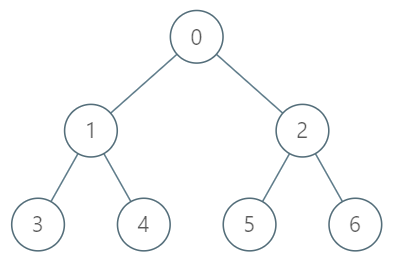["TreeAncestor","getKthAncestor","getKthAncestor","getKthAncestor"]
[[7,[-1,0,0,1,1,2,2]],[3,1],[5,2],[6,3]]

[null,1,0,-1]

TreeAncestor treeAncestor = new TreeAncestor(7, [-1, 0, 0, 1, 1, 2, 2]);

treeAncestor.getKthAncestor(3, 1);  // 返回 1 ，它是 3 的父节点
treeAncestor.getKthAncestor(5, 2);  // 返回 0 ，它是 5 的祖父节点
treeAncestor.getKthAncestor(6, 3);  // 返回 -1 因为不存在满足要求的祖先节点

1 <= k <= n <= 5*10^4
parent == -1 表示编号为 0 的节点是根节点。

0 <= node < n

C++暴力是过不了的，但是js暴力能过。

（1）通过数据结构，采用信息转换的方式，降低单次查询时间

PS：哈希，字典树，状态压缩等，都属于我理解的信息转换，而这题的LCA解法（BFS+DFS+二分）就是属于这一种

（2）通过算法整改解空间，去掉多次查询之间的重复计算

PS：本题的倍增法本质就是动态规划，但是可能动态规划的特征没那么明显

PS：这个思路的前面一半是我比赛时的实际思路，然后我想到带权并查集，然后放弃了这个思路。

PPS：和我 CSU 1515: Sequence 区间DP_csuzhucong的博客-CSDN博客 这个题的做法也比较像。

int ans;

class TreeAncestor {
public:
vector<int>p;
int n;
TreeAncestor(int n, vector<int>& parent) {
p=parent,this->n=n;
for(int i=0;i<n;i++)ans[i]=i;
for(int d=1;d<=200;d++)for(int i=0;i<n;i++)ans[i][d]=ans[i][d-1]>=0?parent[ans[i][d-1]]:-1;
}

int getKthAncestor(int node, int k) {
while(k>200)
{
node=ans[node],k-=200;
if(node==-1)return -1;
}
return ans[node][k];
}
};

int ans;

class TreeAncestor {
public:
vector<int>p;
int n;
TreeAncestor(int n, vector<int>& parent) {
p=parent,this->n=n;
for(int i=0;i<n;i++)ans[i]=parent[i];
for(int d=2;d<18;d++)for(int i=0;i<n;i++)ans[i][d]=ans[i][d-1]>=0?ans[ans[i][d-1]][d-1]:-1;
}

int getKthAncestor(int node, int k) {
for(int i=1;i<18;i++)
{
if(k&(1<<(i-1)))node=ans[node][i];
if(node==-1)return -1;
}
return node;
}
};

PS：

LCA最近公共祖先问题，可以用BFS+DFS+RMQ区间极值来做，也可以用倍增法来做。

# 1486. 数组异或操作

"^" 为按位异或 XOR 运算符。

• 1 <= n <= 1000
• 0 <= start <= 1000
• n == nums.length
class Solution {
public:
int xorOperation(int n, int start) {
if(n==1)return start;
return start^xorOperation(n-1,start+2);
}
};

# 1487. 保证文件名唯一

输入：names = ["pes","fifa","gta","pes(2019)"]

"pes" --> 之前未分配，仍为 "pes"
"fifa" --> 之前未分配，仍为 "fifa"
"gta" --> 之前未分配，仍为 "gta"
"pes(2019)" --> 之前未分配，仍为 "pes(2019)"


输入：names = ["gta","gta(1)","gta","avalon"]

"gta" --> 之前未分配，仍为 "gta"
"gta(1)" --> 之前未分配，仍为 "gta(1)"
"gta" --> 文件名被占用，系统为该名称添加后缀 (k)，由于 "gta(1)" 也被占用，所以 k = 2 。实际创建的文件名为 "gta(2)" 。
"avalon" --> 之前未分配，仍为 "avalon"


输入：names = ["onepiece","onepiece(1)","onepiece(2)","onepiece(3)","onepiece"]



输入：names = ["wano","wano","wano","wano"]

输入：names = ["kaido","kaido(1)","kaido","kaido(1)"]



• 1 <= names.length <= 5 * 10^4
• 1 <= names[i].length <= 20
• names[i] 由小写英文字母、数字和/或圆括号组成。
class Solution {
public:
vector<string> getFolderNames(vector<string>& names) {
unordered_map<string,int>m;
m.reserve(50005);
vector<string>ans;
char cn;
for(int i=0;i<names.size();i++)
{
string s=names[i],s1;
if(m[s]==0)
{
ans.push_back(s);
m[s]=1;
continue;
}
for(int i=m[s];i<50005;i++)
{
sprintf(cn,"%d",i);
s1=s+"("+cn+")";
if(m[s1]==0)
{
ans.push_back(s1);
m[s]=i+1;
m[s1]=1;
break;
}
}
}
return ans;
}
};

PS:

["kaido","kaido(1)","kaido","kaido(1)","kaido(2)"]
["kaido","kaido(1)","kaido(2)","kaido(1)(1)","kaido(2)(1)"]

s和s1都要存入map，改完AC

# 1496. 判断路径是否相交

1 <= path.length <= 10^4
path 仅由 {'N', 'S', 'E', 'W} 中的字符组成

C++代码：

class Solution {
public:
bool isPathCrossing(string path) {
int ns=0,ew=0;
map<pair<int,int>,int>m;
m[make_pair(ns,ew)]=1;
for(int i=0;path[i];i++)
{
if(path[i]=='N')ns++;
if(path[i]=='S')ns--;
if(path[i]=='E')ew++;
if(path[i]=='W')ew--;
if(m[make_pair(ns,ew)])return true;
m[make_pair(ns,ew)]=1;
}
return false;
}
};

C代码：

typedef struct {
int key;
int value;
UT_hash_handle hh;
} Hash;
Hash *hash = NULL;

// 增加
{
Hash *s = NULL;
s = (Hash *)malloc(sizeof(Hash));
s->key = key;
s->value = value;
}
// 查找
int find(int key)
{
Hash *s = NULL;
HASH_FIND_INT(hash, &key, s);
if (s != NULL) {
// 查找到结果
return 1;
} else {
return 0;
}
}
// 删除
void delete (Hash *s)
{
HASH_DEL(hash, s);
free(s);
s = NULL;
}
//清空
void clearAll()
{
Hash *s,*tmp;
HASH_ITER(hh, hash, s, tmp){
delete(s);
}
}

bool isPathCrossing(char * path){
int ns=0,ew=0;
for(int i=0;path[i];i++)
{
if(path[i]=='N')ns++;
if(path[i]=='S')ns--;
if(path[i]=='E')ew++;
if(path[i]=='W')ew--;
if(find(ns*10000+ew))
{
clearAll();
return true;
}
}
clearAll();
return false;
}

04-2853
08-1065
06-2594
05-14114
08-0934
03-121112
07-03634
02-0593
12-131193
06-23491
06-072424
06-22309
12-021276
06-212223
06-27205
05-31158
10-30340
12-07210

### “相关推荐”对你有帮助么？

•非常没帮助
•没帮助
•一般
•有帮助
•非常有帮助被折叠的  条评论 为什么被折叠?到【灌水乐园】发言csuzhucong

¥2 ¥4 ¥6 ¥10 ¥20余额支付 (余额：-- )扫码支付获取中扫码支付点击重新获取扫码支付1.余额是钱包充值的虚拟货币，按照1:1的比例进行支付金额的抵扣。
2.余额无法直接购买下载，可以购买VIP、C币套餐、付费专栏及课程。余额充值